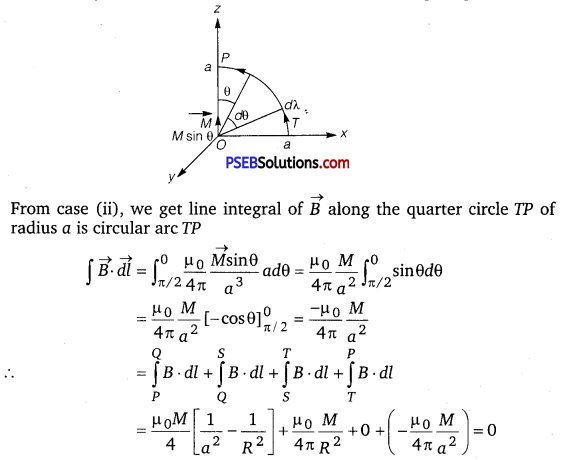# PSEB 12th Class Physics Important Questions Chapter 5 Magnetism and Matter

Punjab State Board PSEB 12th Class Physics Important Questions Chapter 5 Magnetism and Matter Important Questions and Answers.

## PSEB 12th Class Physics Important Questions Chapter 5 Magnetism and Matter

Question 1.
What is the angle of dip at a place where the horizontal and vertical components of the earth’s magnetic field are equal?
The angle of dip is given by
θ = tan-1 ($$\frac{B_{V}}{B_{H}}$$)
BV = vertical component of the earth’s magnetic field.
BH = horizontal component of the earth’s magnetic field.
So, as BV = BH
Then, θ = tan-1 (1) = 45°
∴ The angle of dip will be θ = 45°.

Question 2.
The motion of copper plate is damped when it is allowed to oscillate between the two poles of a magnet. What is the cause of this damping?
As the copper plates oscillate in the magnetic field between the two plates of the magnet, there is a continuous change of magnetic flux linked with the pendulum. Due to this, eddy currents are set up in the copper plate which try to oppose the motion of the pendulum according to the Lenz’s law and finally bring it to rest.Question 3.
Relative permeability of a material μr = 0.5. Identify the nature of the magnetic material and write its relation of magnetic susceptibility.
The nature of magnetic material is a diamagnetic.
μr = 1 + χm

Question 4.
Which of the following substances are diamagnetic?
Bi, Al, Na, Cu, Ca and Ni
Diamagnetic substances are (i) Bi (ii) Cu.

Question 5.
The susceptibility of a magnetic material is -4.2 × 10-6. Name the type of magnetic material, it represents.
Negative susceptibility represents diamagnetic substance.

Question 6.
What are permanent magnets? Give one example.
Substances that retain their attractive property for a long period of time at room temperature are called permanent magnets.
Examples: Those pieces which are made up of steel, alnico, cobalt and ticonal.Question 7.
Why is the core of an electromagnet made of ferromagnetic materials?
Ferromagnetic material has a high retentivity. So on passing current through windings it gains sufficient magnetism immediately.

Question 8.
From molecular view point, discuss the temperature dependence of susceptibility for diamagnetism, paramagnetism and ferromagnetism. (NCERT Exemplar)
Diamagnetism is due to orbital motion of electrons developing magnetic moments opposite to applied field and hence is not much affected by temperature.

Paramagnetism and ferromagnetism is due to alignments of atomic magnetic moments in the direction of the applied field. As temperature increases, this alignment is disturbed and hence susceptibilities of both decrease as temperature increases.

Question 9.
A ball of superconducting material is dipped in liquid nitrogen and placed near a bar magnet.
(i) In which direction will it move?
(ii) What will be the direction of its magnetic moment? (NCERT Exemplar)
When a diamagnetic material is dipped in liquid nitrogen, it again behaves as a diamagnetic material. Thus, superconducting material will again behave as a diamagnetic material. When this diamagnetic material is placed near a bar magnet, it will be feebly magnetised opposite to the direction of magnetising field.

(i) Thus, it will be repelled.
(ii) Also its direction of magnetic moment will be opposite to the direction of magnetic field of magnet.Question 10.
Consider the plane S formed by the dipole axis and the axis of earth. Let P be point on the magnetic equator and in S. Let Q be the point of intersection of the geographical and magnetic equators. Obtain the declination and dip angles at P and Q. (NCERT Exemplar)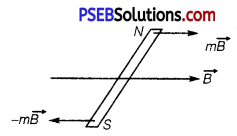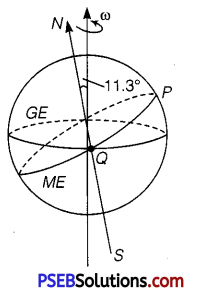(i) P is in S (needle will point both north)
Declination = 0
P is also on magnetic equator.
∴ Dip = 0

(ii) Q is on magnetic equator.
∴ Dip = 0
But declination = 11.3.

Question 1.
Explain the following:
(i) Why do magnetic field lines form continuous closed loops?
(ii) Why are the field lines repelled (expelled) when a
diamagnetic material is placed in an external uniform magnetic field?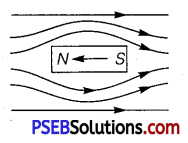(i) Magnetic lines of force form continuous closed loops because a magnet is always a dipole and as a result, the net magnetic flux of a magnet is always zero.
(ii) When a diamagnetic substance is placed in an external magnetic field, a feeble magnetism is induced in opposite direction. So, magnetic lines of force are repelled.

Question 2.
How does a circular loop carrying current behaves as a magnet?
The current round in the face of the coil is in anti-clockwise direction, then this behaves like a North pole, whereas when it viewed from other scale, then current round in it is in clockwise direction necessarily forming South pole of magnet.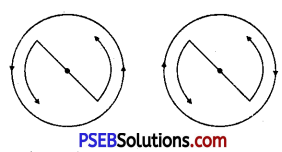Hence, current loop have both magnetic poles and therefore, behaves like a magnetic dipole.Question 3.
Give two points to distinguish between a paramagnetic and diamagnetic substance.

 Paramagnetic substance Diamagnetic substance 1. A paramagnetic substance is feebly attracted by magnet. A diamagnetic substance is feebly repelled by a magnet. 2. For a paramagnetic substance, the intensity of magnetisation has a small positive value. For a diamagnetic substance, the intensity of magnetism has a small negative value.

Question 4.
(a) How is an electromagnet different from a permanent magnet?
Write two properties of a material which makes it suitable for making (i) a permanent magnet, and (ii) an electromagnet.
(a) An electromagnet consists of a core made of a ferromagnetic material placed inside a solenoid. It behaves like a strong magnet when current flows through the solenoid and effectively loses its magnetism when the current is switched off.

A permanent magnet is also made up of a ferromagnetic material but it retains its magnetism at room temperature for a long time after being magnetised one.

(b) Properties of material are as below:
(i) Permanent magnet

• Retentivity and coercivity should be large
• Magnetically hard

(ii) An electromagnet

• Magnetically soft
• Coercivity should be low.

Question 5.
A bar magnet of magnetic moment M and moment of inertia I (about centre, perpendicular to length) is cut into two equal pieces, perpendicular to length. Let T be the period of oscifiations of the original magnet about an axis through the mid-point, perpendicular to length, in a magnetic field B. What would be the similar period T’ for each piece? (NCERT Exemplar)
Given, I = moment of inertia of the bar magnet
m = mass of bar magnet
l = length of magnet about an any passing through its centre and perpendicular to its length
M = magnetic moment of the magnet
B = uniform magnetic field in which magnet is oscillating, we get time period of oscillation is
T = 2π$$\sqrt{\frac{I}{M B}}$$
Here I = $$\frac{m l^{2}}{12}$$
When magnet is cut into two equal pieces, perpendicular to length, then moment of inertia of each piece of magnet about an axis perpendicular to length passing through its centre is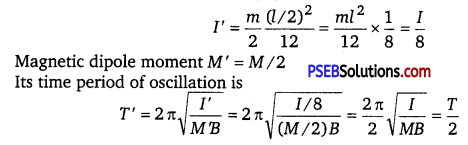Question 6.
A uniform conducting wire of length 12 a and resistance R is wound up as a current carrying coil in the shape of (i) an equilateral triangle of side a; (ii) a square of sides a and, (iii) a regular hexagon of sides a. The coil is connected to a voltage source V0. Find the magnetic moment of the coils in each case. (NCERT Exemplar)

(i) Area of equilateral triangle, A = $$\frac{\sqrt{3}}{4}$$ a2
(ii) Area of square, A = a2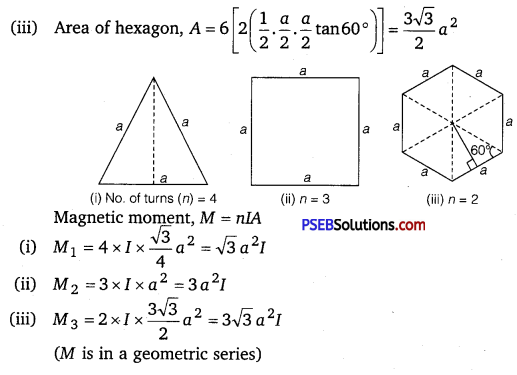Question 1.
Verify the Ampere’s law for magnetic field of a point dipole of dipole moment M = Mk̂. Take C as the closed curve running clockwise along
(i) the z-axis from z a > 0 to z = R,
(ii) along the quarter circle of radius R and centre at the origin in the first quadrant of vz-plane,
(iii) along the x-axis from x = R to x = a, and
(iv) along the quarter circle of radius a and centre at the origin
in the first quadrant of xz-plane (NCERT Exemplar)
From P to Q, every point on the z-axis lies at the axial line of magnetic
dipole of moment $$\vec{M}$$. Magnetic field induction at a point distance z from the magnetic dipole of moment is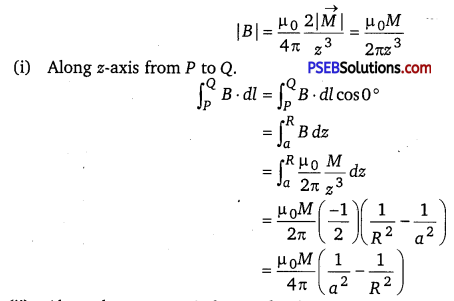(ii) Along the quarter circle QS of radius R as-.given in the figure below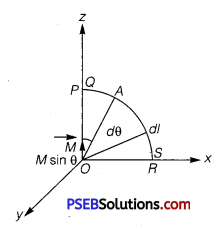The point A lies on the equatorial line of the magnetic dipole of moment M sin0. Magnetic field at point A on the circular arc is
B = $$\frac{\mu_{0}}{4 \pi} \frac{M \sin \theta}{R^{3}}$$ ; $$\overrightarrow{d l}$$ = Rdθ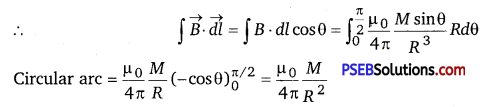(iii) Along x-axis over the path ST, consider the figure given below.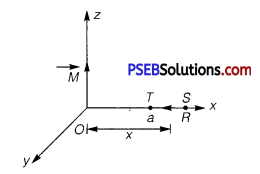From figure, every point lies on the equatorial line of magnetic dipole. Magnetic field induction at a point distance x from the dipole is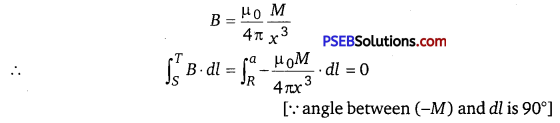(iv) Along the quarter circle TP of radius a. Consider the figure given below